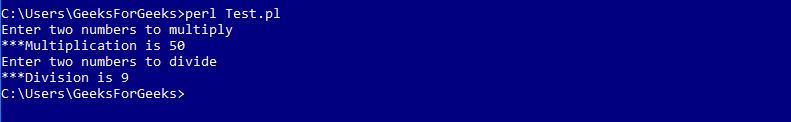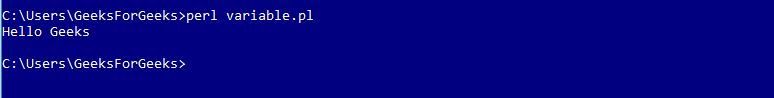Open in App
Not now

# Perl | Modules

• Last Updated : 17 Feb, 2019

A module in Perl is a collection of related subroutines and variables that perform a set of programming tasks. Perl Modules are reusable. Various Perl modules are available on the Comprehensive Perl Archive Network (CPAN). These modules cover a wide range of categories such as network, CGI, XML processing, databases interfacing, etc.

Creating a Perl Module

A modules name must be same as to the name of the Package and should end with .pm extension.

Example : Calculator.pm

 `package` `Calculator; ` ` `  `# Defining sub-routine for Multiplication ` `sub` `multiplication ` `{ ` `    ``# Initializing Variables a & b ` `    ``\$a` `= ``\$_``; ` `    ``\$b` `= ``\$_``; ` `     `  `    ``# Performing the operation ` `    ``\$a` `= ``\$a` `* ``\$b``; ` `     `  `    ``# Function to print the Sum ` `    ``print` `"\n***Multiplication is \$a"``; ` `} ` ` `  `# Defining sub-routine for Division ` `sub` `division ` `{ ` `    ``# Initializing Variables a & b ` `    ``\$a` `= ``\$_``; ` `    ``\$b` `= ``\$_``; ` `     `  `    ``# Performing the operation ` `    ``\$a` `= ``\$a` `/ ``\$b``; ` `     `  `    ``# Function to print the answer ` `    ``print` `"\n***Division is \$a"``; ` `} ` `1; `

Here, the name of the file is “Calculator.pm” stored in the directory Calculator. Notice that 1; is written at the end of the code to return a true value to the interpreter. Perl accepts anything which is true instead of 1

Importing and using a Perl Module

To import this calculator module, we use require or use functions. To access a function or a variable from a module, :: is used. Here is an example demonstrating the same:

Examples: Test.pl

 `#!/usr/bin/perl ` ` `  `# Using the Package 'Calculator' ` `use` `Calculator; ` ` `  `print` `"Enter two numbers to multiply"``; ` ` `  `# Defining values to the variables ` `\$a` `= 5; ` `\$b` `= 10; ` ` `  `# Subroutine call ` `Calculator::multiplication(``\$a``, ``\$b``); ` ` `  `print` `"\nEnter two numbers to divide"``; ` ` `  `# Defining values to the variables ` `\$a` `= 45; ` `\$b` `= 5; ` ` `  `# Subroutine call ` `Calculator::division(``\$a``, ``\$b``); `

Output:Using Variables from modules

Variables from different packages can be used by declaring them before using. Following example demonstrates this
Examples: Message.pm

 `#!/usr/bin/perl ` ` `  `package` `Message; ` ` `  `# Variable Creation ` `\$username``; ` ` `  `# Defining subroutine ` `sub` `Hello ` `{ ` `  ``print` `"Hello \$username\n"``; ` `} ` `1; `

Perl file to access the module is as below
Examples: variable.pl

 `#!/usr/bin/perl ` ` `  `# Using Message.pm package ` `use` `Message; ` ` `  `# Defining value to variable ` `\$Message::username` `= ``"Geeks"``; ` ` `  `# Subroutine call ` `Message::Hello(); `

Output:Using Pre-defined Modules

Perl provides various pre-defined modules which can be used in the Perl programs anytime.
Such as: ‘strict’, ‘warnings’, etc.

Example:

 `#!/usr/bin/perl ` ` `  `use` `strict; ` `use` `warnings; ` ` `  `print``" Hello This program uses Pre-defined Modules"``; `

Output:

`Hello This program uses Pre-defined Modules`

My Personal Notes arrow_drop_up
Related Articles